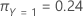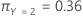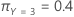# Methods and formulas for misclassification in CART® Classification

Select the method or formula of your choice.

The misclassification table is not present when the splitting method is class probability.

## Count and weighted count

When weights are not used, the counts and the sample sizes are the same.

In the weighted case, the weighted count is the sum of the weights for a category. When you have weights, use the weighted counts to calculate the different weights.

## % Error

In the weighted case, use weighted counts in place of counts.

## Cost

The calculation of cost depends on whether the response variable is binary or multinomial.

Cost = (% Error × Input misclassification cost for class) / 100

### Binary response variable

The following equation gives the cost for the event class:

The following equation gives the cost for the non-event class:

The following equation gives the overall cost for all classes:

### Multinomial response variable

For the multinomial case, the equation extends the formula for the binary response variable to account for all the possible types of misclassifications. For example, for a multinomial response with k classes, the misclassification cost for Y = 1 uses the following equation:

The following equation gives the overall cost for the multinomial case:

For example, consider a response variable with 3 classes and the following misclassification costs:

 Predicted Class Actual class 1 2 3 1 0.0 4.1 3.2 2 5.6 0.0 1.1 3 0.4 0.9 0.0

Then, consider that the following table gives the error percentages:

 Predicted Class Actual class 1 2 3 1 N/A 1% 0.5% 2 1.4% N/A 2.1% 3 5% 1.2% N/A

Finally, consider that the classes of the response variable have the following prior probabilities:

•••The following equations give the costs associated with the misclassification for each class in the response variable:

The following equation gives the overall cost:

By using this site you agree to the use of cookies for analytics and personalized content.  Read our policy